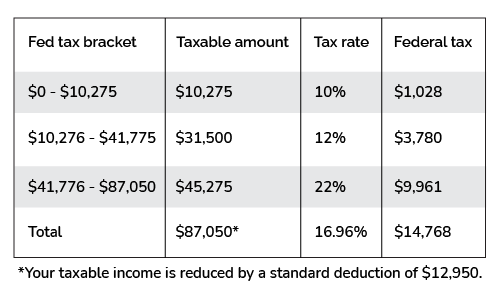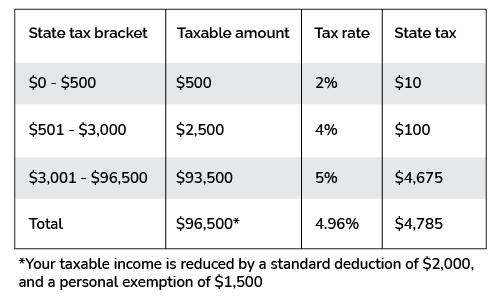# Alabama Tax Calculator

Created by Tibor Pál, PhD candidate and Arturo Barrantes
Reviewed by Dominik Czernia, PhD and Adena Benn
Based on research by
Tax Foundation
Last updated: Dec 27, 2022

The handy Alabama tax calculator (can be used as a paycheck or payroll calculator for Alabama) helps you estimate how much of your adjusted gross income (AGI) will go to taxes. In this article, we will explain how to calculate income tax in Alabama state, including detailed information about tax brackets, state taxes, deductions, and effective tax rates.

Does any of this sound like cryptography to you? Do not worry. Keep reading, and we promise you will be able to calculate the Alabama income tax rate yourself.

## Which are the Alabama taxes?

The taxes a citizen must pay in Alabama include the state income tax, the local income tax, and the general ones: the FICA tax and the federal income tax.

Tax type / Filing status

Single

Married & Jointly

Married & Separately

FICA

7.65%: Social security (6.2%) + medicare (1.45%) taxes

Federal income tax

Progressive tax brackets from 10% to 37%

Deductions in USD

12,950

25,900

12,950

19,400

State income tax

Progressive tax brackets from 2% to 5%

Jurisdiction

Bessemer

Birmingham

Macon County

Local tax

2%

1%

1%

1%

The Alabama paycheck calculator already includes all the specifics indicated above; however, to clarify everything, we will include the following table: tax brackets for the Alabama state income tax. If you need more information about the federal income tax, you should check the tax bracket calculator.

Single filers Alabama tax brackets 2022

Tax rate

Taxable income bracket

Tax owed

Standard deduction

Personal exemption

2%

$0 to$500

2% of taxable income

$2,500 if AGI is below$23,500. To be reduced by $25 for every additional$500 of AGI, not to fall below $2,000.$1,500

4%

$501 to$3,000

$10 + 4% of the amount over$500

5%

$3,001 or more$110 + 5% of the amount over $3,000 Married filing jointly Alabama tax brackets 2022 Tax rate Taxable income bracket Tax owed Standard deduction Personal exemption 2%$0 to $1000 2% of taxable income$7,500 if AGI is below $23,500. To be reduced by$175 for every additional $500 of AGI, not to fall below$4,000.

$3,000 4%$1001 to $6000$20 + 4% of the amount over $1000 5%$6001 or more

$220 + 5% of the amount over$6000

## How do I calculate Alabama income tax?

Before we start, we need a set of information: Your taxing jurisdiction and your filing status as indicated in the Alabama income tax rate table we shared above.

1. Suppose you are a single filer with an AGI of $100,000 living in Gadsden and want to know your 2022 total income tax. Let's start with your FICA, which will be: $\small100,000 \times 7.65 \%$. 2. Now, it is time for your federal income tax. After considering the 2022 deductions that we showed above, your taxable income is$87,050 (that is because $\small 100,000 - 12,950 = \87,050$). Consequently, considering the federal tax progressive income, you pay $4,807.50 plus 22% of the amount over$41,775, which equals a total federal tax of $14,768. Let's explain that a bit more: try the Alabama income tax calculator and click the $\mathrm{\small Summary \ table \ only}$ text (next to the Display option), then click the "Add federal income tax brackets" option. You will see the following table below the summary table:Federal tax distribution of a single filler with AGI$100,000

Notice you reach the 22% bracket, but your income is only partially taxed at that percentage.

1. Let's continue calculating the Alabama state income tax. Change the display to $\mathrm{\small Add \ state \ income \ tax \ brackets}$ in the Alabama tax calculator. Be sure to select the "State tax brackets" display mode. Regarding calculations, follow the next steps to see how to get it:
• Subtract the deductions and exemptions. That means we have an AGI of $\small 100,000 - 2000 - 1500$. Notice we subtract $2000 because tax filler AGI is well above the$23,500 threshold as indicated in the "Single filers Alabama tax brackets 2022". Consequently, we get $96,500 of taxable income. • According to the bottom table, since we are in the$3001 - $96,500 bracket, we must pay$110 plus 5% of the amount over $3,000 as taxes. In other words, $\small 110 + (96,500-3000)\times 5 \%$ which equals$4,785. See the following table from the $\mathrm{\small Add \ state \ income \ tax \ brackets}$ display mode as a summary:Alabama state tax distribution of a single filler with AGI $100,000 1. The next step is to calculate the local income tax. We live in Gadsden; thus, we have to pay a 2% tax rate of$2,000.
2. Finally, we sum all the income tax: $\small 7,650 +14,768 + 4,785 + 2,000 = 29,203$. The total income tax is $29,203, and the total income after tax is$70,797.

In addition, you can estimate your take-home pay for a selected pay frequency by using our tool as the Alabama payroll calculator. For example, if you want to see your monthly take-home pay, you need to select monthly pay frequency, resulting in estimated monthly take-home pay of $5,899.75 in the considered example. ## How to calculate Alabama state income tax for a married couple? You can use the Alabama income tax calculator or do as follows: 1. Suppose you are married, filing jointly, and making$80,000 annually, with no children. Subtract 7.65% of the adjusted gross income (AGI). That will go to your FICA.
2. Locate your marginal tax rate. After deductions ($25,900), we have a marginal tax rate of 12% on $80,000 - $25,900 =$54,100, but remember, the marginal tax rate is only the last bracket we fit in. In this case, you will be taxed progressively: 10% up to $20,550, equivalent to$2055, and the rest at 12%. Thus, we must pay $2,055 plus 12% of the amount over$20,550 (the last bracket), or $6,081. 3. Obtain the Alabama state income tax: Because of your AGI, your standard deduction is$4000 and a couple-exemption of $3000. Hence, your taxable income becomes$73,000. The state income tax is $220 plus 5% of the amount over$6000, which equals $3570 4. Supposing you live in Bessemer, the local income tax is 1% of your AGI, meaning$800.
5. Subtract from your AGI all the taxes stated above. We get an income after tax of $16,571. On the other hand, in the $\mathrm{\small advanced \ mode}$ of the Alabama tax calculator, you can add the number of dependents you have. There you can also include the percentage of your retirement contributions and any itemized deduction you would like to consider. Finally, if you are considering moving to California and/or Texas, we recommend you check their respective tax calculators: ## FAQ ### Does Alabama have state income tax? Yes, Alabama state includes three different brackets of 2%, 4%, and 5% to a set of two types of filers: single filing and married filing jointly. They also include a standard deduction of$2,500 for singles, $7,500 for a couple, and exemptions of$1,500 and $3,000, respectively. ### What is the effective tax rate? The effective tax rate is the percentage of your income that you end up paying in taxes. It considers all federal and state taxes with their particular deductions/exemptions. It differs from the marginal tax rate, which is the last tax bracket you fit in after deductions. ### Is there any local income tax rate in Alabama? Yes. Alabama taxes accordingly to four different jurisdictions: Gadsden, Bessemer, Birmingham, and Macon County, with rates of 2%, 1%, 1%, and 1% accordingly. ### How much tax do I owe if I earn$27,000 in Alabama?

The estimated total annual tax is $4,935. You can find it with the below steps: 1. Subtract the pre-tax deduction:$12,950 to estimate adjusted gross income (AGI): $14,050. Calculate your federal tax rate (12% AGI):$1,481.
2. Subtract the state tax deduction and personal exemption: $2,325 +$1,500. The taxable income is $23,175, and the state income tax is$1,119.
3. Determine local tax depending on tax jurisdiction. For example, in Bessemer, you pay (1% AGI): $270. 4. Calculate FICA tax (7.65% AGI). It is$2,065.5.
5. Sum all taxes above: $4,935. ## Disclaimer You should consider the Alabama tax calculator as a model for financial approximation. All figures and balances are estimates based on the data you provided in the specifications and are not exhaustive, despite our best effort. In addition, the calculator potentially omits information relevant to determining the exact income tax figures. For these reasons, we created the calculator for instructional purposes only. Still, if you experience a relevant drawback or encounter any inaccuracy, we are always pleased to receive helpful feedback and advice. Tibor Pál, PhD candidate and Arturo Barrantes Adjusted Gross Income (AGI)$
/year
Pay frequency
Daily
Year of income
2022
Single
Tax jurisdiction
Bessemer
Results
Display...
summary table only
People also viewed…

### BMR - Harris-Benedict equation

Harris-Benedict calculator uses one of the three most popular BMR formulas. Knowing your BMR (basal metabolic weight) may help you make important decisions about your diet and lifestyle.

### Exit rate

Our exit rate calculator helps us calculate how often visitors exit your website from a particular page.

### Price to book ratio

The price-to-Book Ratio Calculator calculates a ratio that compares the company's market price with the book value.